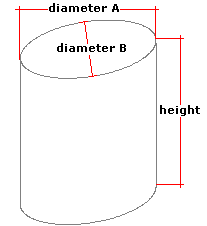Spike's Calculators

# Vertical Elliptical Cylinder TankCalculate a vertical cylinder tank's volume, capacity, and surface area.
The elliptical cylinder tank looks like a squashed cylinder. This calculator uses inches for measurements.

##### Approximate weight of Fuel or Water
```one US gallon of water = 8.34 pounds.
one US gallon of diesel fuel = 7.1 pounds
one US gallon of gas = 6 pounds
```

### Vertical Elliptical Tank

Diameter A in
Diameter B in
Height of the Tank in
Liquid Weight-Pounds per US Gallon lb/gal US

#### Results:

###### Tank Volume
In Cubic Inches in³
In Cubic Feet ft³
Cubic Metres
###### Tank Capacity
In US Gallons gal US
In Imperial Gallons gal Imperial
In Litres L
###### Liquid Weight and Surface Area
Weight of Liquid lbs
Surface Area in Square Inches in²
In Square Feet ft²
In Square Millimetres mm²
In Square Metres

#### Calculator

1. enter diameter A of the tank in inches
2. diameter B of the tank in inches
3. the height of the tank in inches
4. the weight of the liquid in pounds per US gallon

#### Results

1. the volume of the vertical elliptical tank in cubic inches
2. the volume in cubic feet
3. the volume in cubic metres
4. the tank capacity in US gallons
5. the capacity in Imperial gallons
6. the capacity in litres
7. full tank liquid weight based on lbs/gal US
8. the surface area of the tank in square inches
9. the surface area in square feet
10. the surface area in square millimetres
11. the surface area in square metres

##### Formula
```V = 𝝅HWL/4
where V is the volume
𝝅 = 3.14159265
H is the height of the tank
W half of diameter B
L half of diameter A
```
##### Conversions
```one cubic inch (in³) = 0.000578703703703704 cubic feet (ft³)
one cubic inch (in³) = 0.000016387064 cubic metres (m³)
one cubic inch (in³) = 0.00432900432900432 gallons (gal) US
one cubic inch (in³) = 0.00360465014990905 gallons (gal) Imperial
one cubic inch (in³) = 0.016387064 litres (L)
```

### Ellipse - Vertical Tank/Container#### Filled